Courses

# Solutions of Refraction of Light (Page No- 221) - Physics By Lakhmir Singh, Class 10 Class 10 Notes | EduRev

## Class 10 : Solutions of Refraction of Light (Page No- 221) - Physics By Lakhmir Singh, Class 10 Class 10 Notes | EduRev

The document Solutions of Refraction of Light (Page No- 221) - Physics By Lakhmir Singh, Class 10 Class 10 Notes | EduRev is a part of the Class 10 Course Class 10 Physics Solutions By Lakhmir Singh & Manjit Kaur.
All you need of Class 10 at this link: Class 10

Question 39: Which of the following diagrams shows the ray of light refracted correctly ?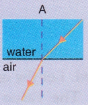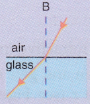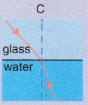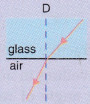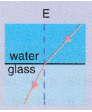Solution :
E.

Question 40: A vertical ray of light strikes the horizontal surface of some water :
(a) What is the angle of incidence ?
(b) What is the angle of refraction ?

Solution : (a) 00
(b) 00

Question 41: How is the reflection of light ray from a plane mirror different from the refraction of light ray as it enters a block of glass ?

Solution : The angle of reflection is equal to the angle of incidence but the angle of refraction is not equal to the angle of incidence.

Question 42: How does the light have to enter the glass :
(a) to produce a large amount of bending ?
(b) for no refraction to happen ?

Solution : (a) Obliquely; making a large angle of incidence.
(b) Perpendicular to the glass surface.

Question 43: (a) How can you bend light away from the normal ?
(b) How must light travel out of a substance if it is not going to be refracted ?

Solution : (a) By making the light enter from a denser medium to a rarer medium.
(b) Incidence should be at right angle to the surface of substance.

Question 44: Draw and complete the following diagrams to show what happens to the beams of light as they enter the glass block and then leave it :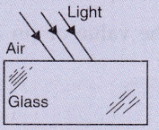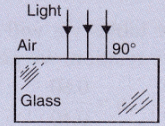Solution :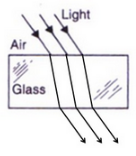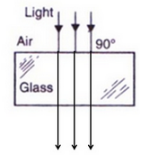Question 45: Why does a beam of light bend when it enters glass at an angle ? Why does it not bend if it enters the glass at right angles ?

Solution : A beam of light bends when it enters glass at an angle. This is due to refraction of light. It does not bend if it enters the glass at right angles because no refraction will occur in this case, the angle of incidence in this case is zero and angle of refraction is also zero.

Offer running on EduRev: Apply code STAYHOME200 to get INR 200 off on our premium plan EduRev Infinity!

94 docs

,

,

,

,

,

,

,

,

,

,

,

,

,

,

,

,

,

,

,

,

,

,

,

,

;